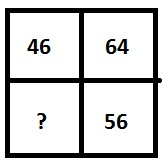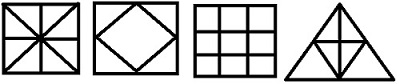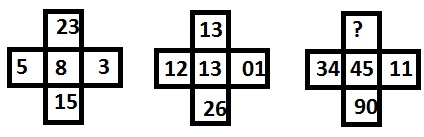# Reasoning - Missing Characters

Inserting a missing figure problems are generally asked in various competitive exams, which mainly consist of a figure divided into various regions. In between these regions, there will be numbers, letters or it may be the combination of letters and numbers following certain sequence. They are in a series inside the given figure. The question may contain single figure or combination of two or more than two figures. The question figure may be any geometrical figure such as rectangle, triangle, circle or other any type of unusual figure to have set of numbers or letters in it. The figure may be divided into many parts according to logic and sequence behind the question. In question figure, the numbers or letters given will be changing in each step by following certain sequence or logic. Reader has to analyze sequence or number or logic and to choose correct answer from given options.

In this type of questions, a single figure will be given which consists of letters or numbers inside it following certain sequence or logic as explained by following example.

Example − 1Options

A − 83

B − 54

C − 65

D − 60

Explanation − In this example, the question figure has four regions inside which there are numbers which are interchanging their position in next step so 56 may be written as 65 to replace question mark.

This type of question may consist of a figure divided into many parts to have numbers or letters in it as shown below,And so many.

Example − 2Options

A − 150

B − 145

C − 165

D − 162

Explanation − The numbers at the bottom row are obtained as (28 - 10) × 10 = 180, (29 - 17) × 11 = 132, (3524) × 14 = 154, therefore missing number is (54 - 36) × 9 = 162.

In type of figure, the question will consist of two or more than two figures of which 1st one or two figures giving information about sequence or logic of question, reader has to analyze them and choose the correct answer as explain by the following example,

Example − 3Options

A − 36

B − 40

C − 45

D − None of these